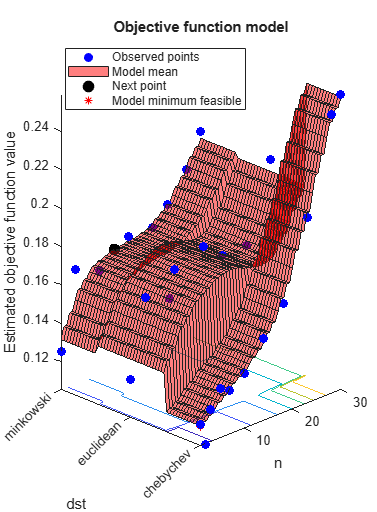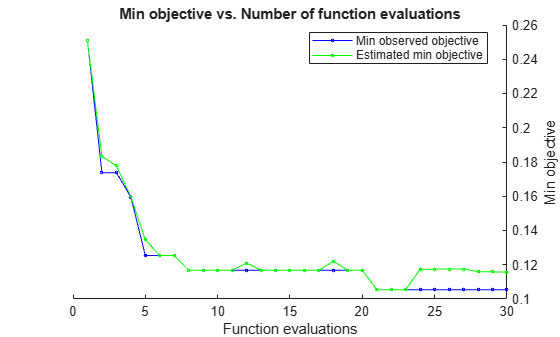bestPoint

Best point in a Bayesian optimization according to a criterion

Description

example

x = bestPoint(results) returns the best feasible point in the Bayesian model results according to the default criterion 'min-visited-upper-confidence-interval'.

example

x = bestPoint(results,Name,Value) modifies the best point using name-value pairs.

example

[x,CriterionValue] = bestPoint(___), for any previous syntax, also returns the value of the criterion at x.

example

[x,CriterionValue,iteration] = bestPoint(___) also returns the iteration number at which the best point was returned. Applies when the Criterion name-value pair is 'min-observed', 'min-visited-mean', or the default 'min-visited-upper-confidence-interval'.

Examples

collapse all

This example shows how to obtain the best point of an optimized classifier.

Optimize a KNN classifier for the ionosphere data, meaning find parameters that minimize the cross-validation loss. Minimize over nearest-neighborhood sizes from 1 to 30, and over the distance functions 'chebychev', 'euclidean', and 'minkowski'.

For reproducibility, set the random seed, and set the AcquisitionFunctionName option to 'expected-improvement-plus'.

rng(11)
num = optimizableVariable('n',[1,30],'Type','integer');
dst = optimizableVariable('dst',{'chebychev','euclidean','minkowski'},'Type','categorical');
c = cvpartition(351,'Kfold',5);
fun = @(x)kfoldLoss(fitcknn(X,Y,'CVPartition',c,'NumNeighbors',x.n,...
'Distance',char(x.dst),'NSMethod','exhaustive'));
results = bayesopt(fun,[num,dst],'Verbose',0,...
'AcquisitionFunctionName','expected-improvement-plus');Obtain the best point according to the default 'min-visited-upper-confidence-interval' criterion.

x = bestPoint(results)
x=1×2 table
n       dst
_    _________

1    chebychev

The lowest estimated cross-validation loss occurs for one nearest neighbor and 'chebychev' distance.

Careful examination of the objective function model plot shows a point with two nearest neighbors and 'chebychev' distance that has a lower objective function value. Find this point using a different criterion.

x = bestPoint(results,'Criterion','min-observed')
x=1×2 table
n       dst
_    _________

2    chebychev

Also find the minimum observed objective function value, and the iteration number at which it was observed.

[x,CriterionValue,iteration] = bestPoint(results,'Criterion','min-observed')
x=1×2 table
n       dst
_    _________

2    chebychev

CriterionValue = 0.1054
iteration = 21

Input Arguments

collapse all

Bayesian optimization results, specified as a BayesianOptimization object.

Name-Value Arguments

Specify optional comma-separated pairs of Name,Value arguments. Name is the argument name and Value is the corresponding value. Name must appear inside quotes. You can specify several name and value pair arguments in any order as Name1,Value1,...,NameN,ValueN.

Example: x = bestPoint(results,'Criterion','min-observed')

Best point criterion, specified as the comma-separated pair consisting of 'Criterion' and a criterion name. The names are case-insensitive, do not require - characters, and require only enough characters to make the name uniquely distinguishable.

Criterion NameMeaning
'min-observed'x is the feasible point with minimum observed objective.
'min-mean'x is the feasible point where the objective model mean is minimized.
'min-upper-confidence-interval'x is the feasible point minimizing an upper confidence interval of the objective model. See alpha.
'min-visited-mean'x is the feasible point where the objective model mean is minimized among the visited points.
'min-visited-upper-confidence-interval'x is the feasible point minimizing an upper confidence interval of the objective model among the visited points. See alpha.

Example: 'Criterion','min-visited-mean'

Probability that the modeled objective mean exceeds CriterionValue, specified as the comma-separated pair consisting of 'alpha' and a scalar between 0 and 1. alpha relates to the 'min-upper-confidence-interval' and 'min-visited-upper-confidence-interval' Criterion values. The definition for the upper confidence interval is the value Y where

P(meanQ(fun(x)) > Y) = alpha,

where fun is the objective function, and the mean is calculated with respect to the posterior distribution Q.

Example: 'alpha',0.05

Data Types: double

Output Arguments

collapse all

Best point, returned as a 1-by-D table, where D is the number of variables. The meaning of “best” is with respect to Criterion.

Value of criterion, returned as a real scalar. The value depends on the setting of the Criterion name-value pair, which has a default value of 'min-visited-upper-confidence-interval'.

Criterion NameMeaning
'min-observed'Minimum observed objective.
'min-mean'Minimum of model mean.
'min-upper-confidence-interval'Value Y satisfying the equation P(meanQ(fun(x)) > Y) = alpha.
'min-visited-mean'Minimum of observed model mean.
'min-visited-upper-confidence-interval'Value Y satisfying the equation P(meanQ(fun(x)) > Y) = alpha among observed points.

Iteration number at which best point was observed, returned as a positive integer. The best point is defined by CriterionValue.